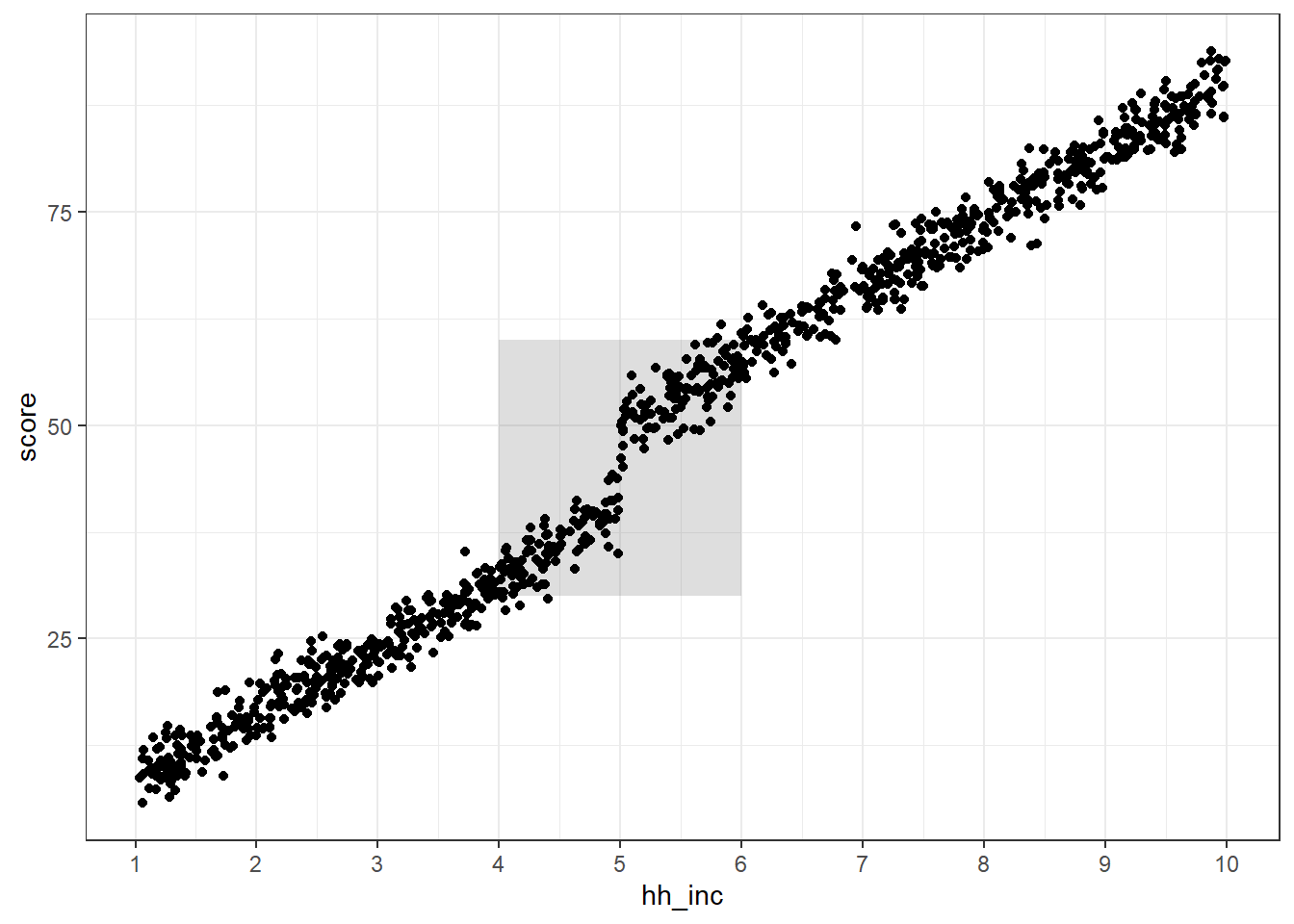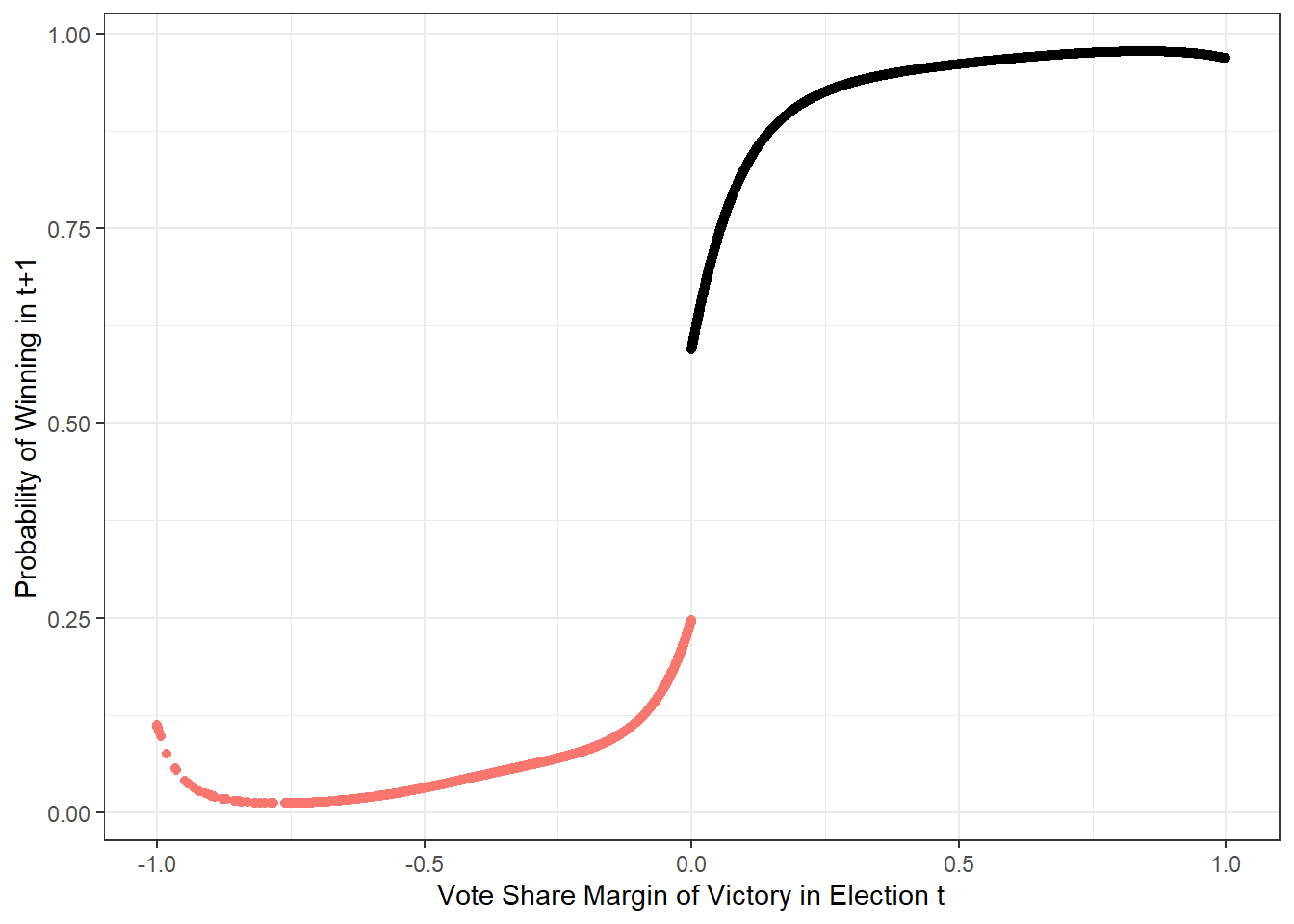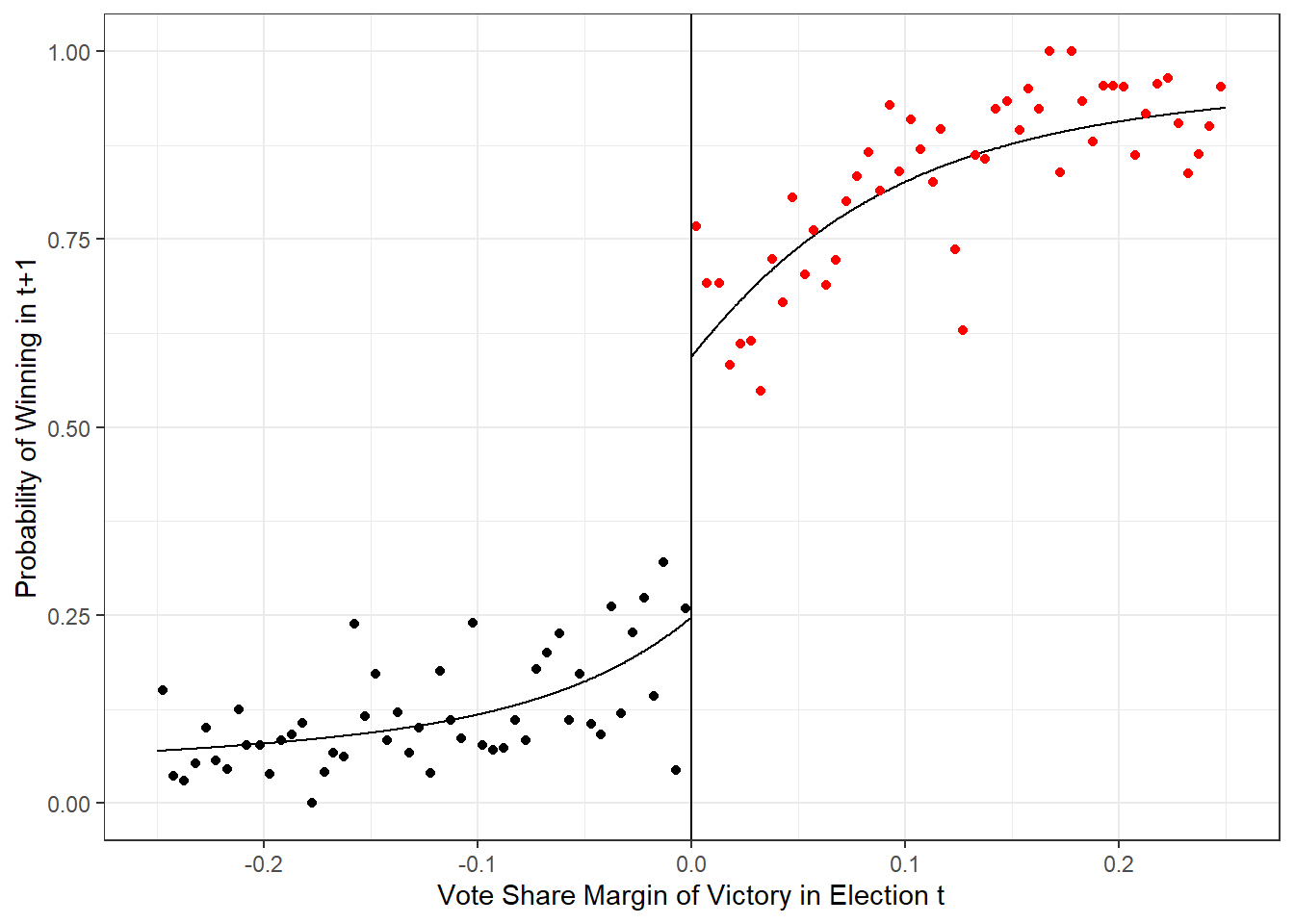## Purpose

This ‘analysis’ is purely a recreational attempt to deepen my own understanding of Regression Discontinuity. I’m going to use a freely available public data set to try and reproduce some of the main results of David Lee’s 2001 paper, The electoral advantage to incumbency and voters’ valuation of politicians’ experience: A regression discontinuity analysis of elections to the U.S. House. I have provided a link to a pdf copy of this paper (and a few other relevant papers) in the Prerequisites section.

The empirical problem that Lee is trying to solve in this particular paper is isolating the causal effect of incumbency on the likelihood of winning a congressional election. This is tricky because in order to be an incumbent one must have won the previous election. So, in this case, there are lots of unobservables that are potentially tangled up with the “incumbency” effect. That is, if we observe that an incumbent candidate wins the election in time $$t+1$$, how much of that win is attributable specifically to incumbency versus other unobservables (like charisma or being really good at campaigning)?

The Regression Discontinuity approach is Lee’s proposal for isolating the effect of incumbency. Specifically, he isolates the “incumbency effect” by comparing outcomes of elections in time $$t+1$$ for candidates that just barely lost an election in time $$t$$ (those who failed to become incumbents) and those that just barely won their elections in time $$t$$ (those that became incumbents in $$t+1$$). Conceptually, candidates who just won/just lost in election $$t$$ should be similar enough in all respects EXCEPT for incumbency…such that if there is a significant difference between the success rate for these two groups in $$t+1$$ it can be attributed to the “incumbency advantage.”

## Prerequisites

Here are the packages and libraries that this module relies on:

library(rddtools)
library(dplyr)
library(ggplot2)

I’m also basing most of this post on the follower NBER paper by David Lee:

Lee 2001, "The electoral advantage to incumbency and voters’ valuation of politicians’ experience: A regression discontinuity analysis of elections to the U.S. House

A related paper was published in 2008 in the Journal of Econometrics. It is interesting but not quite as straightforward in terms of trying to reproduce the empirics:

Lee 2008, “Randomized experiments from non-random selection in U.S. House elections”

## Methods Background

I would like to keep this section short and conceptually focused. There are two ‘overview’ resources I recommend on RDD:

The idea behind RDD is that, sometimes in quasi-treatment-control set ups, there are algorithmic rules that define who receives the treatment. A rather classic example is income-based rules for determining who gets some type of assistance. Think about public schools where some kids get free or subsidized lunch because of their poverty status. If one were interested in the impact of free or reduced price lunch on educational outcomes, this effect would be hard to estimate because it is tangled up with poverty status (all of the kids receiving free lunch are poor and all of the kids not receiving free lunch are less poor).

RDD would propose to help isolate the causal impact of free/reduced price lunch by examining educational outcomes for students in a small neighborhood around the cut-off. The ideas is that students just a little to left and those just a little to the right of the cut-off are similarly poor…but experience different levels of the treatment (those just below the income threshold get the treatment while those just above do not).

Here’s a totally fake example where test scores scale linearly with household income but are discontinuous at a hypothetical income cut-off below which students qualify for free lunch:

hh_inc<- runif(1000,min=1,max=10)
eps <- rnorm(1000,0,2)
df <- data.frame(hh_inc=hh_inc) %>% mutate(mean.score=ifelse(hh_inc<=5,hh_inc*8,10+(hh_inc*8)),
score=mean.score+eps)

ggplot() +
geom_rect(data=data.frame(xmin=c(4),xmax=c(6),ymin=c(30),ymax=c(60)),
aes(xmin=xmin,xmax=xmax,ymin=ymin,ymax=ymax),alpha=0.2) +
geom_point(data=df,aes(x=hh_inc,y=score)) +theme_bw() + scale_x_continuous(breaks=c(1:10))## Data

The first thing to notice here is that the data provided to the rddtools package are pretty sparse. If one refers to any of the Tables in Lee (2001) one will see many variables - like candidate political years of experience or electoral experience - that do not exist in this simple 2-column data frame.

data(house)
head(house)
##         x      y
## 1  0.1049 0.5810
## 2  0.1393 0.4611
## 3 -0.0736 0.5434
## 4  0.0868 0.5846
## 5  0.3994 0.5803
## 6  0.1681 0.6244

## Lee (2001) Figure 2

This section looks at trying to reproduce Figure 2a from Lee (2001). If I’m being totally honest I don’t actually understand Lee’s Figure 2 very well. The points are “local averages”, the line is the “logit fit”, and the vertical axis is probability space. My data set has x and y which need to be transformed into probability values. The “logit fit” for making this transformation is pretty straightforward. I’m not sure what the “local average” probability value is. I’m going to compute an empirical probability and see how it looks.

The first problem that I ran into here is that the data I have do not have an indicator for whether the candidate won or lost in the t+1 election. The data frame include x which are the margins of victory in the time t election and y which is purported to be margin of victory in the t+1 election but are obviously the vote shares from the t+1 election (note that there are no negative values for y).

To get around this I’m going to apply a sensible yet imperfect assumption: I’ll create a variable called win that equals 1 if y>.5 and 0 otherwise. This is imperfect because the potential presence of 3rd party candidates means that an incumbent or challenger could win the t+1 election with less than 50% of the vote…but considering that the observed outcome of the t+1 election was not included in the data, this seems like an ok hack.

house <- house %>% mutate(win=ifelse(y>0.5,1,0))

Note that Figure 2a plots the probability of running and winning election t+1 against the vote share margin of victory for the time t election. In order to reproduce this, I need to construct a probability that the candidate wins in t+1 from the data frame:

winners <- house %>% filter(x>0)
losers <- house %>% filter(x<=0)
logit.win <- glm(win~x+I(x^2)+I(x^3)+I(x^4),data=winners,family='binomial')
logit.lose <- glm(win~x+I(x^2)+I(x^3)+I(x^4),data=losers,family='binomial')
winners$win.hat <- predict(logit.win,type='response') losers$win.hat <- predict(logit.lose,type='response')

Now I’ll plot these predicted probabilities against the vote share margin of victory:

ggplot(house) + geom_point(data=winners,aes(x=x,y=win.hat)) + geom_point(data=losers,aes(x=x,y=win.hat,color="red")) +
theme_bw() + theme(legend.position="none") +
ylab("Probability of Winning in t+1") + xlab("Vote Share Margin of Victory in Election t")This looks kinda like Figure 2a…but Lee formatted his plots a little differently: He binned the observations and just displayed observations within 0.25 of the cutoff on either side.

#bin data
losers.binned <- losers %>% mutate(bin=cut(x,breaks=seq(from=-1,to=0,by=0.005),include.lowest=T))
winners.binned <- winners %>% mutate(bin=cut(x,breaks=seq(from=0,to=1,by=0.005),include.lowest=T))

# take the "local average"...the mean y value for each bin
losers.binned <- losers.binned %>% dplyr::group_by(bin) %>%
dplyr::summarise(nbin=n(),x=mean(x),y=sum(win)/nbin)

winners.binned <- winners.binned %>% dplyr::group_by(bin) %>%
dplyr::summarise(nbin=n(),x=mean(x),y=sum(win)/nbin)

ggplot(house) + geom_line(data=winners,aes(x=x,y=win.hat)) + geom_line(data=losers,aes(x=x,y=win.hat)) +  geom_point(data=losers.binned,aes(x=x,y=y)) +
geom_point(data=winners.binned,aes(x=x,y=y),color="red") +
geom_vline(xintercept=0) + xlim(c(-0.25,0.25)) + theme_bw() +
ylab("Probability of Winning in t+1") + xlab("Vote Share Margin of Victory in Election t") +
theme(legend.position="none")## Table 2

The attempts to reproduce Figure 2a from Lee (2001) were a little unsatisfactory…but since I don’t have the full data set there were some hacks I had to employ to get close. Table 2a has some regression results that I might be able to reproduce with the limited data set.

Note that I’m not totally clear on where the binning of observations come into Lee’s analysis. That is, I’m not sure if he’s binning the data before or after estimating his regression equations. I’m going to estimate the regression equations using the full data sample (not the smoothed binned averages) and see how it plays.

Here I’m going to estimate a linear model predicting vote share in election $$t+1$$ using vote share margin of victory in election $$t$$ as the predictor variable. Following Lee’s approach I use a 4th order polynomial and estimate the regression separately for:

1. winners of the time $$t$$ election, and
2. losers of the time $$t$$ election
incumbents <- house %>% filter(x>0)
challenger <- house %>% filter(x<=0)

d_1 <- lm(y~x+I(x^2)+I(x^3)+I(x^4),data=incumbents)
d_0 <- lm(y~x+I(x^2)+I(x^3)+I(x^4),data=challenger)

Next, note that as x –> 0 from the right the predicted vote share value is 0.530 with a standard error of 0.0093. We can compare this with Lee’s estimate of 0.531 and a standard error of 0.008

summary(d_1)
##
## Call:
## lm(formula = y ~ x + I(x^2) + I(x^3) + I(x^4), data = incumbents)
##
## Residuals:
##      Min       1Q   Median       3Q      Max
## -0.87482 -0.06933 -0.00436  0.07627  0.46649
##
## Coefficients:
##              Estimate Std. Error t value Pr(>|t|)
## (Intercept)  0.530758   0.009358  56.720  < 2e-16 ***
## x            0.543110   0.142671   3.807 0.000143 ***
## I(x^2)      -0.704775   0.616563  -1.143 0.253080
## I(x^3)       1.236156   0.959914   1.288 0.197901
## I(x^4)      -0.730429   0.481050  -1.518 0.128995
## ---
## Signif. codes:  0 '***' 0.001 '**' 0.01 '*' 0.05 '.' 0.1 ' ' 1
##
## Residual standard error: 0.1413 on 3813 degrees of freedom
## Multiple R-squared:  0.3796, Adjusted R-squared:  0.3789
## F-statistic: 583.2 on 4 and 3813 DF,  p-value: < 2.2e-16

Next, note that as x –> 0 from the left, the predicted vote share value is 0.454 with a standard error of 0.0091. We can compare this to Lee’s estimate of 0.454 with a standard error 0f 0.008.

summary(d_0)
##
## Call:
## lm(formula = y ~ x + I(x^2) + I(x^3) + I(x^4), data = challenger)
##
## Residuals:
##      Min       1Q   Median       3Q      Max
## -0.44427 -0.04827  0.00660  0.06049  0.71516
##
## Coefficients:
##             Estimate Std. Error t value Pr(>|t|)
## (Intercept)  0.45417    0.00913  49.743  < 2e-16 ***
## x            0.52360    0.14835   3.529 0.000423 ***
## I(x^2)       1.52922    0.69553   2.199 0.027987 *
## I(x^3)       4.22015    1.17213   3.600 0.000323 ***
## I(x^4)       3.04520    0.62343   4.885  1.1e-06 ***
## ---
## Signif. codes:  0 '***' 0.001 '**' 0.01 '*' 0.05 '.' 0.1 ' ' 1
##
## Residual standard error: 0.1273 on 2735 degrees of freedom
## Multiple R-squared:  0.2774, Adjusted R-squared:  0.2763
## F-statistic: 262.5 on 4 and 2735 DF,  p-value: < 2.2e-16

## Deeper Dive

Regression Discontinuity is all about the details. It’s one thing to make cute little plots and say, “yeah, things look totally discontinuous at that break point” it’s quite another to demonstrate that all the assumptions of the technique are satisfied and the effect being hypothesized is actually identifiable. Here is a good resource for the ‘in-the-weeds’ stuff. Spoiler alert, it’s pretty dense with a lot of math:

Hanh, Todd, and Van der Klaauw (2001), "Identification and Estimation of Treatment Effects with a Regression Discontinuity Design

It is also worth noting that the causal effect of interest in a RDD can be estimated non-parametrically. Josh Angrist has this to say about non-parametric estimation:

The validity of RD estimates of causal effects based on (6.1.4) or (6.1.6) [regression equations] turns on polynomial models provide an adequate description of $$E[Y_{0i}|x_i]$$. If not, then what looks like a jump due to treatment might simply be an unaccounted for nonlinearity in the counterfactual conditional mean function….To reduce the likelihood of such mistakes we can look only at the data in a neighborhood around the discontinuity, say the interval $$[x_0 - \delta, x_0 + \delta]$$ for some small positive number $$\delta$$. Then we have:

$$E[Y_i|x_0 - \delta < x_i < x_0] ~= E[Y_{0i}|x_i=x_0]$$ $$E[Y_i|x_0 < x_i < x_0 + \delta] ~= E[Y_{1i}|x_i=x_0]$$

so that

$$lim (\delta -> 0) E[Y_i|x_0 <= x_i <= x_0 + \delta]-E[Y_i|x_0 - \delta < x_i < x_0] = E[Y_{1i}-Y_{0i}|x_i=x_0]$$

In other words, comparison of average outcomes in a small enough neighborhood to the left and right of $$x_0$$ estimate the treatment effect in a way that does not depend on the correct specification of a model for $$E[Y_{0i}|x_i]$$. Moreover, the validity of this nonparametric estimation strategy does not turn on the constant effects assumption, $$Y_{1i} - Y_{0i} = \rho$$; the estimand in (6.1.7) is the average causal effect…